# Lesson Notes By Weeks and Term - Primary 2

TERM: 1st Term

WEEK: 5

CLASS: Primary 2

AGE: 7 years

DURATION: 5 periods of 40 minutes each

DATE:

SUBJECT: Mathematics

SPECIFIC OBJECTIVES: At the end of the lesson, the pupils should be able to

1. Solve Addition and subtraction of 2-digit numbers and one-digit numbers.
2. Solve addition and subtraction problems with 2-digit numbers and multiples of 10.

INSTRUCTIONAL TECHNIQUES: Explanation, question and answer, demonstration, practical

INSTRUCTIONAL MATERIALS: Printed tens, bottle tops and place value table per learner,

 PRESENTATION TEACHER’S ACTIVITY PUPIL’S ACTIVITY STEP 1MENTAL MATHS The teacher begins the lesson with some mental calculationsWhat number comes just after1 4   2 3    3 9    4 15  5 19  6 187 168 299 1710 99 Pupils respond and participate STEP 2CONCEPTDEVELOPMENT The teacher• Writes 24 + 3 = on the board.• Gives the learners a few minutes to work on the problem individually.• Asks learners to discuss their strategies and answers with the person sitting next to them.• Lets some pairs present their work on the board.• For corrections shows how to work in a place value table as follows:• Uses base ten kits to show the calculation on the place value table.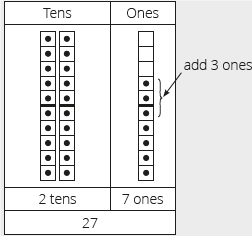In the first put 2 tens and 4 ones (bottle tops) in the place value table. Add 3 ones by putting an extra 3 bottle tops in the ones place on the place value table.With the learners work out how many ones there are now. There are 7 ones altogether.The answer to 24 + 3 is 27, which is 2 tens and 7 ones.Look at the tens place – that did not change – no tens were added.This calculation is preparing the learners for the column method which will be introduced in the next unit.• Writes 95 – 2 = on the board.• Uses base ten kits to show the calculation on the place value table.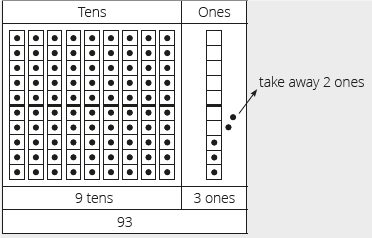In the first step, use tens and bottle tops to show the number 95 in the place value table– 9 tens and 5 ones.You need to subtract 2.Take away 2 ones (remove two bottle tops) as shown in the diagram above.• With the learners finds out how much is left over. There are 3 ones left over.So 95 – 2 = 93, the answer is 9 tens and 3 ones, which is 93.Look at the tens place – that did not change – no tens were subtracted.This calculation is preparing the learners for the column method which will be introduced in the next unit. CLASS ACTIVITYThe teacherIn this activity we solve 2-digit and single digit problems using a number line.We will use the same problems as activity 1.• Draws a number line from 20 to 30 on the board as shown below.• Writes 24 + 3 = on the board.• Discusses with the learners how to solve this, if you use a number line.• Shows the learners how we move forwards 3 spaces from 24 to 27 on the number line.So 24 + 3 = 27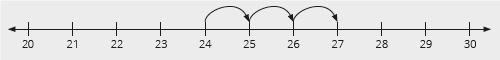• Writes 95 – 2 = on the board• Draws a number line from 90 to 100 on the board as shown below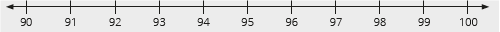• Discusses with the learners how to solve this.• Shows the learners how we move backwards 2 spaces from 95 to 97 on the number line.So 95 – 2 = 93.ACTVITY IIThe teacherIn this activity you will be solving a word problem with your learners.Word problems are a challenging topic for learners. However, if we teach them properly, learners will be better prepared and able to solve them.• Reads the following word problem to your learners. Sarah has 10 sweets. Venita gives her another 5 sweets. How many sweets does she have altogether? The first step is to understand the question properly.When solving word problems there are four main steps:Step 1: Understand the problem,Step 2: Devise a plan,Step 3: Carry out the plan,Step 4: Look back and check what you have done. • In step 1, we write the word problem on the board. Next, we read the problem. Then, welet the learners read the problem themselves in order to understand the problem.• After that we underline the numbers. These are 10 and 5.• Lets learners discuss which operation they will use, addition or subtraction to solvethis problem.Learners write a number sentence in their classwork books that expresses the solutionto the problem.• Checks who wrote the correct number sentence and who did not.• Writes a number sentence as 10 + 5 = 15 on the chalkboard for confirmation of thecorrect solution.• Lets learners work on the problem using any strategies they want to use.• You could also display the answer using a place value table. As correction, present the diagram below using base ten kits to show the problem in a diagrammatic form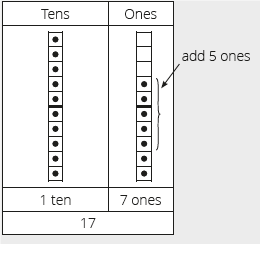Pupils pay attention and participate STEP 3CLASS-WORK NOTE: Learners should use their own base ten kits while they work through this activity.Note also that the solutions below in the lesson plans show the base ten kit solutions.Learners should use their base ten kits to find the solutions but they must record theirnumeric answers only using the place value tables.Calculate using your base ten kit. Record your answers in the place value tables.a 43 + 6 = ___b.28 – 7 = ___c 31 + 5 = ___d.36 – 4 = ___e Sam has 26 marbles. He loses 6. How many marbles does he have left? Pupils attempt their class work STEP 4HOME-WORK Calculate using your base ten kit. Record your answers in the place value tables.a 23 + 5 = ___b 47 – 7 = ___c Tom has 32 pencils.George gives him another 4 pencils.How many pencils does he have altogether? The pupils writes it in their homework book STEP 5SUMMARY The teacher summarizes by reminding the pupils that in this lesson we solved solved addition and subtraction problems. In the questions we added and subtracted one-digit numbers to/from two-digit numbers. She marks their class works, makes corrections where necessary and commends them positively

 PRESENTATION TEACHER’S ACTIVITY PUPIL’S ACTIVITY STEP 1MENTAL MATHS The teacher begins the lesson with some mental calculationsWhat is 10 more than1 52 3  3 7  4 0  5 30  What is 10 less than6 507 308 209 1910 70 Pupils respond and participate STEP 2CONCEPTDEVELOPMENT The teacher• Draws a place value table on the board and display the number 25 in it, using your base ten kit.• Using the diagram interactively with the class, revise the steps to solve the question25 + 10 = .• Asks: How would you solve this problem?• Shows the learners 20 as 2 tens and place them in the tens place.• Asks: What are we going to add to 25? (10)Yes, we will add 10 which is 1 ten. Place the 1 ten next to 20 as shown in the diagram below.• Asks: How many 10s are there altogether (3 tens which is 30)• Asks: How many ones there are? (5)• Add the tens and ones together. What is the answer? (35)• Lets learners be aware that they do not change anything in the ones column.• Write 94 – 20 = on the board• Draw a place value table on the board and display the number 94 in it, using yourbase ten it.• Using the diagram interactively with the class, revise the steps to solve the question94 – 20 = .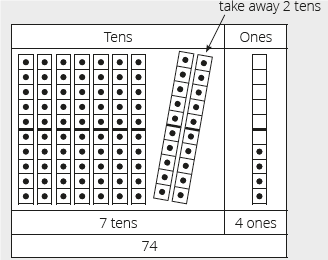• Asks: How many tens and how many ones make up 94? (9 tens and 4 ones)• Places 9 ten frames and 4 ones on the place value table as shown above. As you place the printed tens on the place value table count in 10s until 9 tens (90) and in ones until 4 ones (4) has been put in the place value table.• Asks: What are we going to subtract from 94? (20)Yes, we will subtract 20 which is 2 tens.• Removes 2 tens from the tens place. As you remove them say I am subtracting20 from 90.• Asks: How many 10s and ones are left? There are 7 tens and 4 ones left, which is 74.• Looks at the ones place – that did not change – no ones were subtracted. ACTIVITY IIThe teacher• Gives each pair of learners a 100 board• Writes the following question on the board: 11 + 10 =• Asks: Where do we find 11 on the number board? Let the learners show you 11. Now we need to add 10 to 11. Discusses with the learners what the answer could be. Shows the learners that to add 10 we can jump vertically to 21.• Repeats these steps with the following examples using the 100 board:• For example:• 42 + 10 = 52• 49 – 10 = 39• 35 + 40 = 75• 53 – 30 = 23• Revisits these calculations using number lines.• Draws the number lines on the board. Asks the learners to explain the number lines.• Asks: What do you observe on the number lines? (Only the numbers of tens have changed. There is no change in the ones. The numbers in ones stay as they are, etc.)• You may select different learners to explain each example.Pupils pay attention and participate STEP 3CLASS-WORK NOTE: Learners should use their own base ten kits while they work through this activity.Note also that the solutions below in the lesson plans show the base ten kit solutions.Learners should use their base ten kits to find the solutions but they must record theirnumeric answers only using the place value tablesCalculate using your base ten kit. Record your answers in the place value tables.a 33 + 10 = ___b 48 – 20 = ___c 21 + 30 = ___d 16 – 10 = ___e Use the number line below to solve this word problem.Thandi has 24 mini cars.His brother gives him another 10.How many mini cars does he have altogether?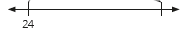Pupils attempt their class work STEP 4HOME-WORK Calculate using your base ten kit. Record your answers in the place value tables.a 44 + 10 = ___b 27 – 10 = __c Use the number line below to solve this word problem.John has 14 sweets.He gives Lilian 10 sweets.How many sweets does he have left? The pupils writes it in their homework book STEP 5SUMMARY The teacher summarizes by reminding the pupils that in today’s lesson we solved addition and subtraction problems involving multiples of 10 and 2-digit numbers. We used number lines, place value tables and base ten kits to solve these problems. She marks their class works, makes corrections where necessary and commends them positively

PERIOD 3: Assessment

 PRESENTATION TEACHER’S ACTIVITY PUPIL’S ACTIVITY STEP 1ORALASSESSMENTS The teacher asks questions on mental maths treated so far Pupils respond and participate STEP 2DISCUSSION The teacher discusses all the methods used by some learners in the oral assessments(some of the questions are solved on the board by the learners) and addresses any misconceptions that may have risen Pupils pay attention and participate STEP 3WRITTEN ASSESSMENTS 1 Complete the place value tables.(Learners only record the numbers in the place value table but check that they use their base ten kits if they need to when they work out how to fill in the table.)a. 16b. 31c. 50d. 35e. 62 2 Complete the following:a 30 + 10 = ____b 50 – 20 = ____c 40 – 30 = ____d 80 + 10 = ____e 100 – 60 = ____ 3 Calculate using your base ten kit. Record the answers in the place value tables.(Learners only record the numbers in the place value table but check that they use their base ten kits if they need to when they work out the answers.)a 24 + 4 =b. 56 – 4 =c. 35 + 10 =d. 76 – 20 =e. 30 + 30 = Pupils attempt their class work STEP 4SUMMARY The teacher marks the written assessments, corrects were necessary and commends the pupils

 PRESENTATION TEACHER’S ACTIVITY PUPIL’S ACTIVITY STEP 1MENTAL MATHS The teacher begins the lesson with some mental calculationsCalculate1 __ – 4 = 22 __ – 3 = 63 __ – 4 = 54 __ – 2 = 65 __ – 7 = 06 __ + 3 = 77 __ + 2 = 78 __ + 7 = 99 __ + 3 = 1010 __ + 0 = 8 Pupils respond and participate STEP 2CONCEPTDEVELOPMENT The teacherREVISES EXCHANGING 10 ONES FOR 1 TEN.• Writes the following questions on the board:a 9 + 4b 8 + 6c 7 + 5d 5 + 9e 12 – 4f 13 – 6g 15 – 6h 17 – 9• Lets learners their 2 ten frames and bottle tops to practice:• addition with carrying (a to d) and• subtraction without borrowing (e to h)• Work through the examples with the class, showing the exchange of ones (revise usingbottle tops as ones). CLASS ACTIVITYThe teacherREVISES BREAKING DOWN A NUMBER INTO TENS AND ONES.• Writes the following questions on the board:• Breaks down the numbers into sums of tens and ones: (Show them using a place value table and base ten kit if necessary – revises using printed tens as tens.)• 25 = __ + __ (20 + 5)• 63 = __ + __ (60 + 3)• 79 = __ + __(70 + 9)• 55 = __ = __(50 + 5)• 38 = __ + __(30 + 8)• Calls some learners up to the board to write up the solutions. ACTIVITY IIThe teacher• Writes the following number sentence on the board: 40 + 30 = __ (70)• Discusses with the learners: We are going to add 40 and 30.• Asks: How do we do this?• Draws a place value table on the board to work with while you explain the calculation.• Asks one learner to come and put 40 onto the place value table using a base ten kit.What will you put onto the table? (he/she must places 4 tens onto the place value table)• Asks: How can we add 30 to the 40? (we add 3 tens to the 40 or 4 tens)• Lets another learner place 3 printed tens into the tens column/place in the placevalue table.The display on the board will now look like this:• Counts the number of tens together with the learners. How many tens are there? (7 tens)How else can we say this? We can say 40 + 30 equals 70.• Writes the following number sentence on the board: 60 – 20 = __ (40)• Discusses with the learners: We are going to subtract 20 from 60.• Asks: How do we do this?• Draws a place value table on the board to work with while you explain the calculation.• Asks one learner to come and put 60 onto the table using a base ten kit. What will you put onto the table? (he/she must places 6 tens onto the place value table)• Asks: How can we subtract 20 from 60? (we take away 2 tens)• Asks a learner to come and show the subtraction of 20. (A learner comes and takes away 2 tens.)The display on the board will now look like this:• Counts the number of tens together with the learners. How many tens are there? (4 tens)How else can we say this? We can say 60 – 20 = 40. Pupils pay attention and participate STEP 3CLASS-WORK NOTE: Note: Learners should work with their base ten kits when they do this activity in class. Theymust use the printed tens and ones (bottle tops) (only if necessary), to reinforce working with numbers broken down into tens and ones.1 Complete the following:a 3 tens + 3 ones = _____b 6 tens + 8 ones = _____c 82 = 80 + _____d 55 = _____  + _____e 90 + _____  = 93f _____  + 6 = 66g  _____ + 9 = 59 2 Complete using tens and ones:a 14 = ___ + ____b 23 = ____ + ____c 32 = ___ + ____d 50 = _____ + ____e 99 = _____ + ___ 3 Calculate using your base ten kit. Record your answers in the place value tables.a 40 + 20 = ____b 80 – 40 = _____ (40) Pupils attempt their class work STEP 4HOME-WORK Complete using tens and ones:1 24 = ____ + ____2 45 = ____ + ____3 80 + _____  = 834 _____  + 6 = 665 _____  + 9 = 596 Bisi has 7 suckers.She buys another 5 suckers.How many does she have altogether? The pupils writes it in their homework book STEP 5SUMMARY The teacher summarizes by reminding the pupils that in today’s lesson we have solved addition and subtraction problems involving multiples of 10 and single-digit numbers. We also revised working with tens and ones – exchanging 10 ones for 1 ten. We also practised breaking down numbers into tens and ones – for example, 34 is 3tens and 4 ones. She marks their class works, makes corrections where necessary and commends them positively

PERIOD 5: Weekly Test/consolidations

TEACHER’S ACTIVITY: The teacher revises all the concepts treated from period 1-4 and gives the pupils follow through exercises, quiz and tests. She marks the exercises, makes corrections and commends the pupils positively.

PUPIL’S ACTIVITY: The pupils work on the worksheets and exercises give by the teacher individually

CONSOLIDATION

NOTE: Learners should use their own base ten kits while they work through this activity.

Solutions of base ten kit displays are shown below.

1 Complete:

a 5 tens + 6 ones = ____

b 64: ___ tens + ___ ones = ___

c 35: ___ tens + ___ ones = ___

d 7 tens and 8 ones = ___

e 90:___tens + ___ ones = ____

a 40 + 30 = ____

b 70 – 40 = ____

c 36 + 3 = ____

d 77 – 6 = ____

e 34 + 20 = ____

f 75 – 20 = ____

3 Complete using tens and ones:

a 45 = ___ + ______

b 83 = ____ + _____

c 52 = ____ + _____

d 80 = _____ + ____

e 69 = _____ + ____

1. Georgina has 27 pencils.

She loses 10 pencils.

How many pencils does she have left?